# 2012 A-level H2 Mathematics (9740) Paper 1 Question 3 Suggested SolutionsAll solutions here are SUGGESTED. Mr. Teng will hold no liability for any errors. Comments are entirely personal opinions.

(i)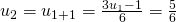(ii)
As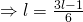(iii)
Let P(n) be the statement “” forWhen.

Thus, P(1) is true.

Assume P(k) is true for some, i.e.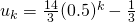To prove P(k+1) is true, i.e.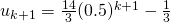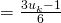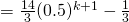Since P(1) is true, by Mathematical Induction, P(k) is trueP(k+1) is true, P(n) is true for allNot readable? Change text.